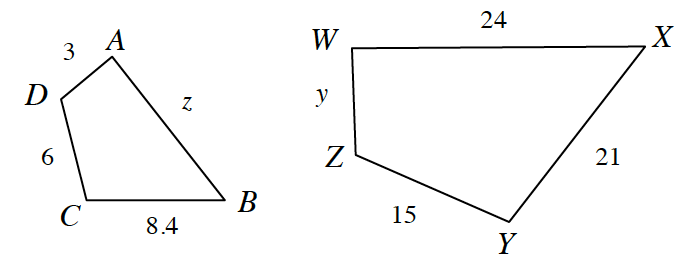### Home > INT2 > Chapter 5 > Lesson 5.1.1 > Problem5-13

5-13.

List a sequence of transformations that demonstrates $ABCD \sim WXYZ$, then solve for $y$ and $z$.What two transformations are needed to move $ABCD$ onto $WXYZ$?

Which sides correspond? Use those sides to write a proportion. For example, $\frac{6}{15}=\frac{z}{24}$.

$z=9.6$